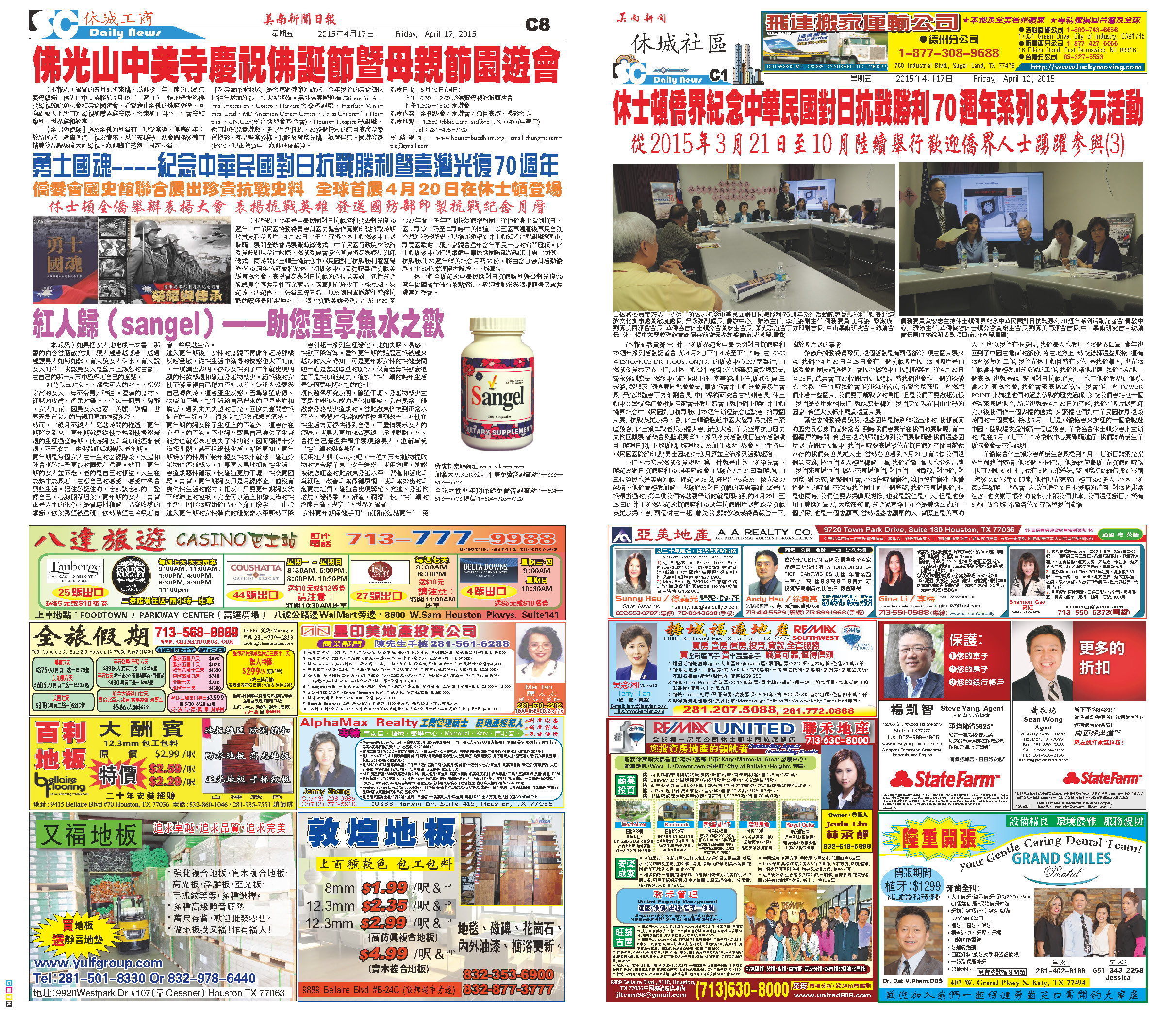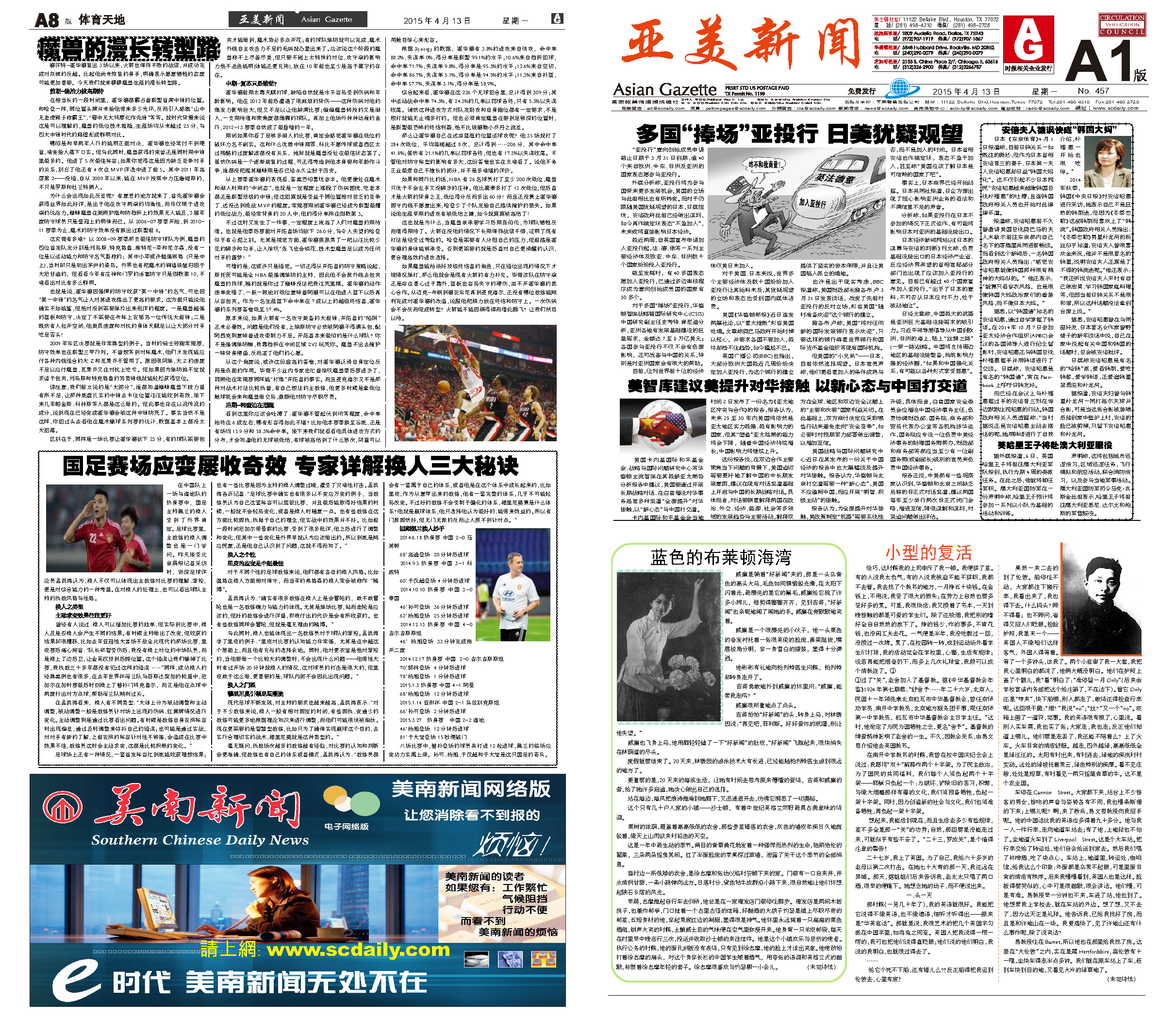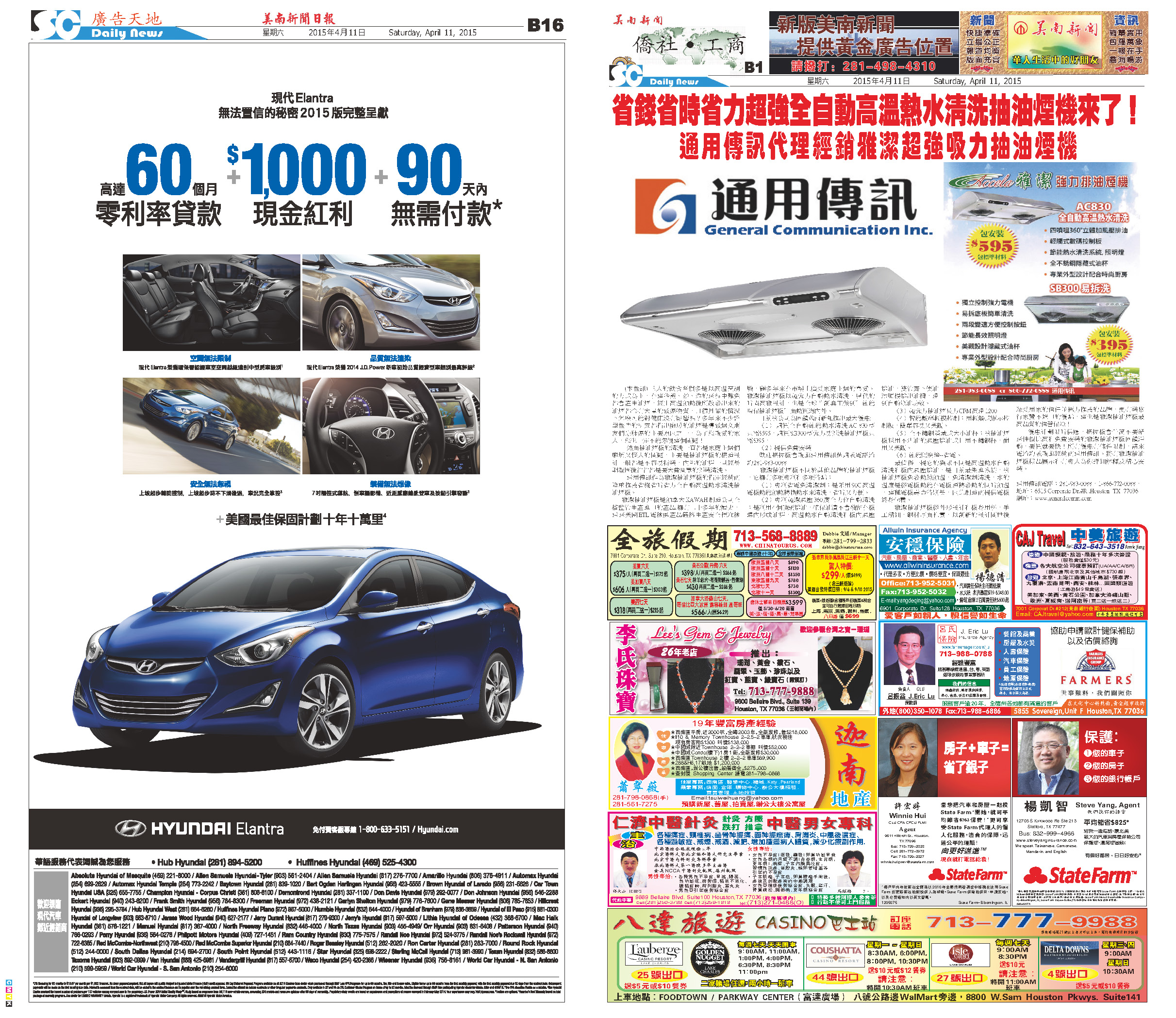150419 Epaper

 A Section B SectionC Section D Section150418 Epaper

 A Section B SectionC Section D Section150417 Epaper

 A Section B SectionC Section D Section150416 Epaper

 A Section B SectionC Section D Section150415 Epaper

 A Section B SectionC Section D Section150413 Epaper

 A Section B SectionC Section D Section150412 Epaper

 A Section B SectionC Section D Section150411 Epaper

 A Section B SectionC Section D Section150409 Epaper

 A Section B SectionC Section D Section150410 Epaper

 A Section B SectionC Section D Section150408 Epaper

 A Section B SectionC Section D Section